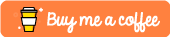# Simple Age and Gender detection using Python and OpenCV

## Introduction

Hello! In this tutorial I will show how to create a simple age and gender detector using Python and OpenCV. 😃

• Python 3

## Creating the python virtual environment

Creating a virtualenv using python 3 is very simple and you don't need to install any modules etc.

``````python3 -m venv env
``````

Then we just need to activate it.

``````source env/bin/activate
``````

This example requires only the opencv-python so we will define this in the "requirements.txt" file.

``````opencv-python
``````

Save it and then install the requirements via:

``````pip install -r requirements.txt
``````

This will install opencv-python into the virtual environment that was created.

The models and weights needed for this can be found via: https://github.com/ethand91/python-gender-age-detect/tree/master/weights

All you need to do is download them and put them into a directory called "weights".

## Creating the Python file

Now we can finally get started writing Python, first we need to import the required modules.

### 1. Importing the modules

``````import cv2
import math
import sys
``````

### 2. Define the model/weight files

Next we need to define and load the models and weights etc.

``````# Defined the model files
FACE_PROTO = "weights/opencv_face_detector.pbtxt"
FACE_MODEL = "weights/opencv_face_detector_uint8.pb"

AGE_PROTO = "weights/age_deploy.prototxt"
AGE_MODEL = "weights/age_net.caffemodel"

GENDER_PROTO = "weights/gender_deploy.prototxt"
GENDER_MODEL = "weights/gender_net.caffemodel"

MODEL_MEAN_VALUES = (78.4263377603, 87.7689143744, 114.895847746)
AGE_LIST = ["(0-2)", "(4-6)", "(8-12)", "(15-20)", "(25-32)", "(38-43)", "(48-53)", "(60-100)"]
GENDER_LIST = ["Male", "Female"]

``````

### 3. Getting the bounding box coordinates

Next we need to get the face coordinates and we also draw a rectangle on the image via the following:

``````def get_face_box (net, frame, conf_threshold = 0.7):
frame_copy = frame.copy()
frame_height = frame_copy.shape
frame_width = frame_copy.shape
blob = cv2.dnn.blobFromImage(frame_copy, 1.0, (300, 300), [104, 117, 123], True, False)

net.setInput(blob)
detections = net.forward()
boxes = []

for i in range(detections.shape):
confidence = detections[0, 0, i, 2]

if confidence > conf_threshold:
x1 = int(detections[0, 0, i, 3] * frame_width)
y1 = int(detections[0, 0, i, 4] * frame_height)
x2 = int(detections[0, 0, i, 5] * frame_width)
y2 = int(detections[0, 0, i, 6] * frame_height)
boxes.append([x1, y1, x2, y2])
cv2.rectangle(frame_copy, (x1, y1), (x2, y2), (0, 255, 0), int(round(frame_height / 150)), 8)

return frame_copy, boxes
``````

### 4. Predicting age and gender

Next we use the following to predict the age and gender of the person, we also draw the age and gender on the image via:

``````def age_gender_detector (input_path):
resized_image = cv2.resize(image, (640, 480))

frame = resized_image.copy()
frame_face, boxes = get_face_box(FACE_NET, frame)

for box in boxes:
face = frame[max(0, box - box_padding):min(box + box_padding, frame.shape - 1), \

blob = cv2.dnn.blobFromImage(face, 1.0, (227, 227), MODEL_MEAN_VALUES, swapRB = False)
GENDER_NET.setInput(blob)
gender_predictions = GENDER_NET.forward()
gender = GENDER_LIST[gender_predictions.argmax()]
print("Gender: {}, conf: {:.3f}".format(gender, gender_predictions.max()))

AGE_NET.setInput(blob)
age_predictions = AGE_NET.forward()
age = AGE_LIST[age_predictions.argmax()]
print("Age: {}, conf: {:.3f}".format(age, age_predictions.max()))

label = "{},{}".format(gender, age)
cv2.putText(frame_face, label, (box, box - 10), cv2.FONT_HERSHEY_SIMPLEX, 0.8, (0, 255, 255), 2, cv2.LINE_AA)

return frame_face
``````

### 5. Writing main

Finally we write the starting point of the program:

``````if __name__ == "__main__":
output = age_gender_detector(sys.argv)
cv2.imwrite("output/output.jpg", output)
cv2.imshow("Output", output)

cv2.waitKey(0)
cv2.destroyAllWindows()
``````

Here we take the file path as argv and predict the age and gender of the people in the image. The output is also written to the output directory (you may need to create this directory before running).

After this the output is shown to the user until the user presses any key. Usage example:

``````python main.py lena.jpg
``````

If all goes well the following should be displayed:Feel free to try it with different images.

Github Repo: https://github.com/ethand91/python-gender-age-detect

Like me work? I post about a variety of topics, if you would like to see more please like and follow me. Also I love coffee.